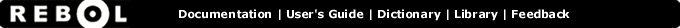# / - Function Summary

## Summary:

Returns the first value divided by the second.

/ value1 value2

## Arguments:

value1 - The value1 argument. (must be: number pair char money time tuple)

value2 - The value2 argument. (must be: number pair char money time tuple)

## Description:

An error will occur if the second value is zero. When dividing values of different datatypes they must be compatible.

 ``` print 123 / 10 12.3```

 ``` print 12.3 / 10 1.23```

 ``` print 1:00 / 60 0:01```

## Related:

* - Returns the first value multiplied by the second.
// - Returns the remainder of first value divided by second.
divide - Returns the first value divided by the second.